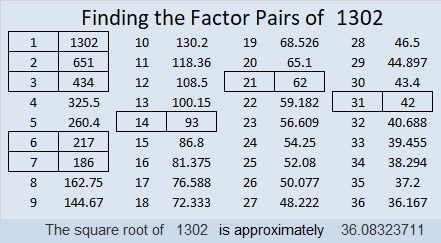# 1302 and Level 1

If you can tell time on an analog clock, then you can solve this puzzle. Go ahead and see how little time it will take you!Print the puzzles or type the solution in this excel file: 10-factors-1302-1310

Here are a few facts about the number 1302:

• 1302 is a composite number.
• Prime factorization: 1302 = 2 × 3 × 7 × 31
• The exponents in the prime factorization are 1, 1, 1, and 1. Adding one to each and multiplying we get (1 + 1)(1 + 1)(1 + 1)(1 + 1) = 2 × 2 × 2 × 2 = 16. Therefore 1302 has exactly 16 factors.
• Factors of 1302: 1, 2, 3, 6, 7, 14, 21, 31, 42, 62, 93, 186, 217, 434, 651, 1302
• Factor pairs: 1302 = 1 × 1302, 2 × 651, 3 × 434, 6 × 217, 7 × 186, 14 × 93, 21 × 62, or 31 × 42
• 1302 has no square factors that allow its square root to be simplified. √1302 ≈ 36.08324This site uses Akismet to reduce spam. Learn how your comment data is processed.